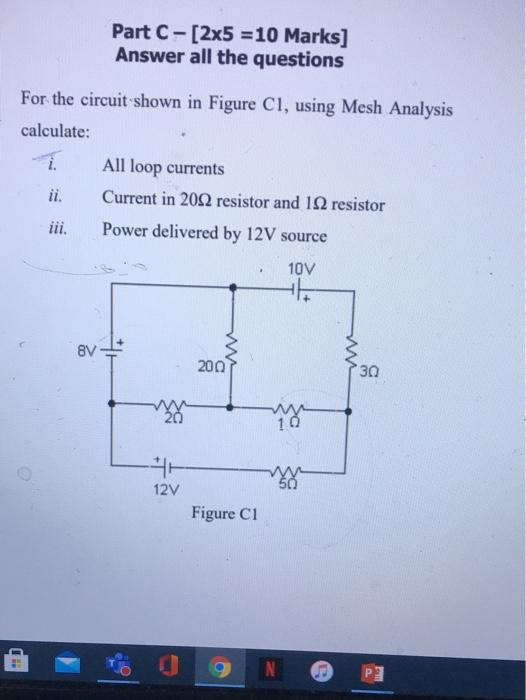# Question Solved1 AnswerPart C-[2x5 = 10 Marks] Answer all the questions For the circuit shown in Figure Cl, using Mesh Analysis calculate: All loop currents Current in 2022 resistor and 19 resistor Power delivered by 12V source iii, 10V . BV 200 30 10 12V Figure C1 N CECAWAQQ The Asker · Electrical EngineeringTranscribed Image Text: Part C-[2x5 = 10 Marks] Answer all the questions For the circuit shown in Figure Cl, using Mesh Analysis calculate: All loop currents Current in 2022 resistor and 19 resistor Power delivered by 12V source iii, 10V . BV 200 30 10 12V Figure C1 N CE
More
Transcribed Image Text: Part C-[2x5 = 10 Marks] Answer all the questions For the circuit shown in Figure Cl, using Mesh Analysis calculate: All loop currents Current in 2022 resistor and 19 resistor Power delivered by 12V source iii, 10V . BV 200 30 10 12V Figure C1 N CE